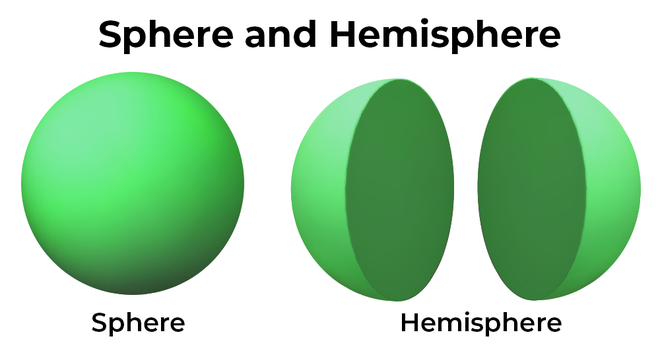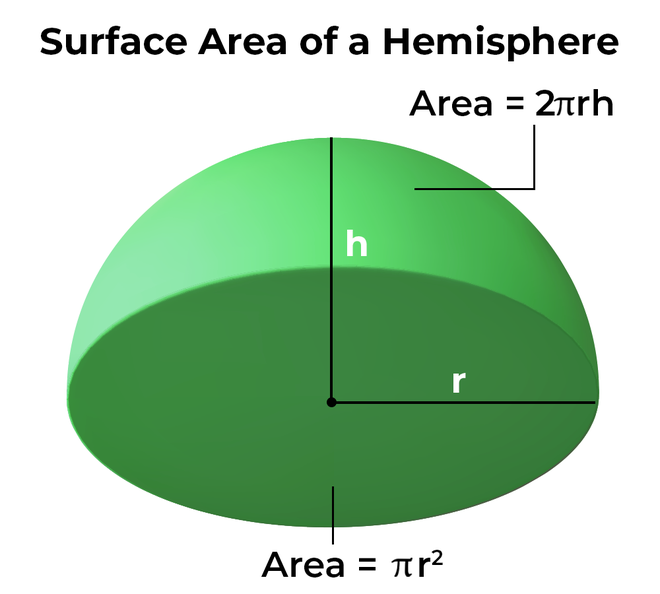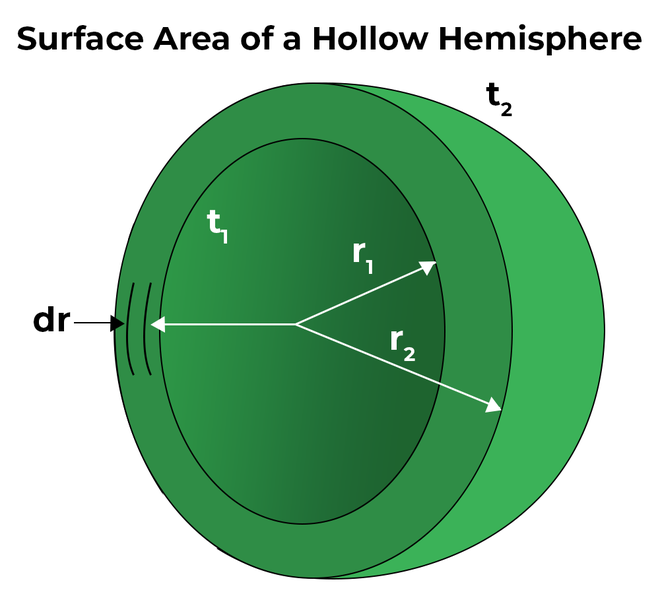Open In App
Related Articles

# Surface Area of a Hemisphere

Hemisphere is a 3D object whose shape and size are half of that of a sphere. The bowl in your kitchen, and the half of a watermelon all are examples of a Hemisphere. A hemisphere can be obtained by cutting a sphere laterally or transversely. The hemisphere so obtained will have the same diameter as the sphere along the direction in which it was cut out from a sphere while the other perpendicular direction will only have half of the diameter i.e. only one radius. Since a hemisphere is a cut-out part of a sphere it has one flat surface and one curved surface, unlike a sphere which has no flat surface and only a curved surface.

In this article, we will learn in detail about the calculation of volume and surface areas of a hemisphere, its derivation, and some solved examples.

## What is a Hemisphere?

A hemisphere is formed when a plane divides a sphere into two equal parts. In other words, a hemisphere is exactly half of a sphere in geometry. It is made up of two parts: “hemi,” meaning half, and “sphere,” which is a three-dimensional mathematical shape for round objects. When a sphere is sliced at the exact centre along its diameter, two equal hemispheres are generated. Therefore, a hemisphere is a three-dimensional geometric object consisting of half of a sphere with one side flat and the other side shaped like a round bowl.Sphere and Hemisphere

### Real-Life Examples of Hemispheres

The examples of hemispheres can be seen in everyday life. For instance, the bowl that we use to eat food from is nothing but a hollow hemisphere. The half-cut shell of the coconut is an example of a hollow hemisphere. When we cut round-shaped fruits like oranges, tamarind, watermelon, etc., the shape of the fruit becomes a solid hemispherical shape. Below shown images are real-life examples of hemispheres.Real-Life

## What is the Surface Area of a Hemisphere?

The surface area of a hemisphere is the total surface area of a hemisphere. The Hemispherical area is defined by two types of hemispheres, the solid hemisphere, and the hollow hemisphere. The surface area can be found in two ways:

• Curved Surface Area of a Hemisphere (CSA).
• Total Surface Area of a Hemisphere (TSA).

## Curved Surface Area of Hemisphere Formula

The curved surface area of the hemisphere formula is defined as the area covered by its curved surface. It is equal to half of the total surface area of a sphere. The formula for the curved surface area of the hemisphere equals two times the product of pi and the square of the radius of the hemisphere.

Curved Surface Area of a Hemisphere = 2πr2

Where,

• π is a constant with the value of 3.14, and
• r is the radius of hemisphere.

### Derivation of the Formula Curved Surface Area of a Hemisphere

Curved Surface Area for Hemisphere is the area of all the curved surfaces of the hemisphere which is only half of the spherically curved surface as the base of the hemisphere is a flat surface that is not curved. Thus,

Curved surface area of a hemisphere = 1/2 × (Curved Surface Area of Sphere)

CSA = 1/2 (4πr2)

CSA = 2πr2

### Base Area of Hemisphere

The base of the hemisphere is in a circular shape, and therefore, the formula for the base area of the hemisphere is equal to the area of a circle.

Base Area of Hemisphere = πr2

## Total Surface Area of a Hemisphere Formula

The total surface area of a hemisphere is defined as the total space covered by the surface of the hemisphere. The total surface area is given by the sum of its curved surface area and base area. The formula for total surface area equals three times the product of the pi (π) and the square of the radius of the hemisphere.Total Surface Area of Hemisphere = 3πr2

Where,

• π is a constant with the value of 3.14, and
• r is the radius of hemisphere.

### Derivation of the Formula Total Surface Area of a Hemisphere

Total surface area for a hemisphere is the sum of the curved surface area of the hemisphere and the area of its circular base since a hemisphere is just half of a sphere with a circular base. Therefore, the total surface area of a hemisphere can be expressed as:

Total Surface Area of a Hemisphere = Curved surface area of Hemisphere + Base area of Hemisphere

⇒ TSA = 2πr2 + πr2

TSA = 3πr2

## Surface Area of a Hollow Hemisphere Formula

The surface area of a hollow hemisphere formula should be first explained in order to understand the formula well. A hollow hemisphere has two diameters as the hollow inner hemisphere creates another smaller diameter. If noted carefully, it can be seen that the surface area of a hollow hemisphere consists of the curved surface area of the bigger hemisphere, the curved surface area of the smaller hemisphere, and the area of the remaining base.

### Derivation of the Formula Surface Area of a Hollow Hemisphere

Let’s break down the areas in order to obtain the surface area of a hollow hemisphere,

• The curved surface area of the outer hemisphere = 2πR2
• The curved surface area of the inner hemisphere = 2πr2
• The base area of the hollow hemisphere = π(R2 – r2)

Therefore, the total surface area of the hemisphere = 2πR2 + 2πr2 + π(R2 – r2)

TSA = 2πR2 + 2πr2 + πR2 – πr2

TSA = 3πR2 + πr2## How to Find the Surface Area of a Hemisphere?

The surface area of a hemisphere can be found by following easy steps based on what type of hemisphere is given. If a solid hemisphere is given, the formula of total surface area and curved surface area can be used based on the requirement, and if a hollow hemisphere is given, the formula for a hollow hemisphere must be used. Following are the steps that can be followed to obtain the surface areas based on the requirement.

### How to Find the Curved Surface Area of a Hemisphere

The formula for the curved surface area of a hemisphere when the given radius is “r” is 2πr2. Below are the steps provided to find the curved surface area of a hemisphere:

• Note down the radius of the hemisphere.
• Put the “r” value in the formula for the curved surface area of a sphere, that is, CSA = 2πr2.
• Present the final answer in square units.

Example: Calculate the curved surface area of a hemisphere radius of 5 m. (Use π = 3.14).

Solution:

We have,

r = 5

Using the formula we get,

CSA = 2πr2

⇒ CSA = 3 (3.14) (5)2

⇒ CSA = 235.5 sq. m

### How to Find the Total Surface Area of a Hemisphere

The formula for the total surface area of a hemisphere when the given radius is “r” is 3πr2. Below are the steps provided to find the curved surface area of a hemisphere:

• Note down the radius of the hemisphere.
• Put the “r” value in the formula for the total surface area of a sphere, that is, TSA = 3πr2.
• Present the final answer in square units.

Example: Calculate the total surface area of a hemisphere diameter of 16cm.

Solution:

We have,

• d = 16cm
• r = 8cm

Using the formula we get,

TSA = 3πr2

⇒ TSA = 3 (3.14) (8)2

⇒ TSA = 602.88 sq. cm.

### How to Find the Surface Area of a Hollow Hemisphere

The formula for the surface area of a hollow hemisphere when the given radius is “r” is 3πR2 + πr2. Below are the steps provided to find the surface area of a hollow hemisphere:

• Note down the radius of the hemisphere.
• Put the “r” value in the formula for the total surface area of a sphere, that is, TSA = 3πR2 + πr2.
• Present the final answer in square units.

## Summary of Surface Area of Hemisphere

A hemisphere is also called a semi-sphere or half a sphere. It is formed when a plane divides a sphere into two equal parts. When a sphere is sliced at the exact centre along its diameter, two equal hemispheres are generated. The surface area of a hemisphere, also known as the total surface area of a hemisphere, is the sum of all the areas of its faces, including the curved surface and the base.

• CSA of Hemisphere = 2πr2

The base area of a hemisphere is the area of the flat surface at the bottom of the hemisphere.

• Base area of Hemisphere = πr2
• Total Surface Area (TSA) of Hemisphere = 3πr2

The surface area of a hollow hemisphere can be calculated using the following formula the sum of the inner surface, an outer surface, and the area of the base which is in the shape of a ring.

• Curved Surface Area of a Hollow Hemisphere =  2π(R2 + r2)
• Total Surface Area of a Hollow Hemisphere = 3πR2 + πr2

Where r is the radius of the hemisphere and in the case of the hollow hemisphere R and r are the outer and inner radius of the hollow hemisphere.

## Solved Example on Surface Area of Hemisphere

Example 1: Calculate the total surface area of a hemisphere radius of 4 m.

Solution:

We have,

r = 4

Using the formula we get,

TSA = 3πr2

⇒ TSA = 3 (3.14) (4)2

⇒ TSA = 150.72 sq. m

Example 2: Calculate the radius of a hemisphere if its total surface area is 200 sq. m.

Solution:

We have,

A = 200

Using the formula we get,

A = 3πr2

⇒  r2 = A/3π

⇒  r2 = 200/3 (3.14)

⇒  r = 4.60 m

Example 3: Calculate the radius of a hemisphere if its total surface area is 350 sq. m.

Solution:

We have,

A = 200

Using the formula we get,

A = 3πr2

⇒  r2 = A/3π

⇒  r2 = 350/3 (3.14)

⇒  r = 6.09 m

Example 4: Calculate the curved surface area of a hemisphere radius of 4 m.

Solution:

We have,

r = 4

Using the formula we get,

CSA = 2πr2

⇒ CSA = 3 (3.14) (4)2

⇒ CSA = 150.72 sq. m

Example 5: Calculate the radius of a hemisphere if its curved surface area is 790 sq. m.

Solution:

We have,

A = 790

Using the formula we get,

A = 2πr2

⇒ r2 = A/2π

⇒ r2 = 350/2 (3.14)

⇒ r = 7.46 m

## FAQs on Surface Area of a Hemisphere

### Q1: What is a Hemisphere?

A hemisphere is a three-dimensional shape that is half of a sphere. It has a curved surface and a flat base.

### Q2: What Units are used to Measure the Surface Area of a Hemisphere?

The surface area of a hemisphere can be measured in square units, such as square centimeters (cm²) or square meters (m²).

### Q3: What is the Lateral Surface Area of a Hemisphere?

The lateral surface area of hemisphere is another name for the curved surface area of hemisphere and the formula for curved surface area of a hemisphere is 2πr2.

### Q4: What is the Area of the Base of a Hemisphere?

The base area of hemisphere has circular shape and therefore, the area of circle will be used to find the base area of hemisphere. Hence, base area becomes πr2.

### Q5: What is the Formula for the Total Surface Area of a Hemisphere?

The formula for the total surface area of a hemisphere is 3πr2, where r is the radius of the hemisphere.

### Q6: How to find the Surface Area of a Hemisphere?

The formula for total surface area of a hemisphere when the given radius is “r” is 3πr2. Below are the steps provided to find curved surface area of a hemisphere:

• Note down the radius of the hemisphere.
• Put the “r” value in the formula for total surface area of sphere, that is, TSA = 3πr2.
• Present the final answer in square units.

### Q7: Explain the difference between the Curved Surface Area of a Hemisphere and the Surface Area of a Hemisphere.

The curved surface area of hemisphere is only the area covered by the curved surface and the formula for curved surface area of hemisphere is 2πr2. While the surface area of a hemisphere is the sum of the curved surface area and the base area of hemisphere, therefore, the formula for TSA becomes 3πr2.